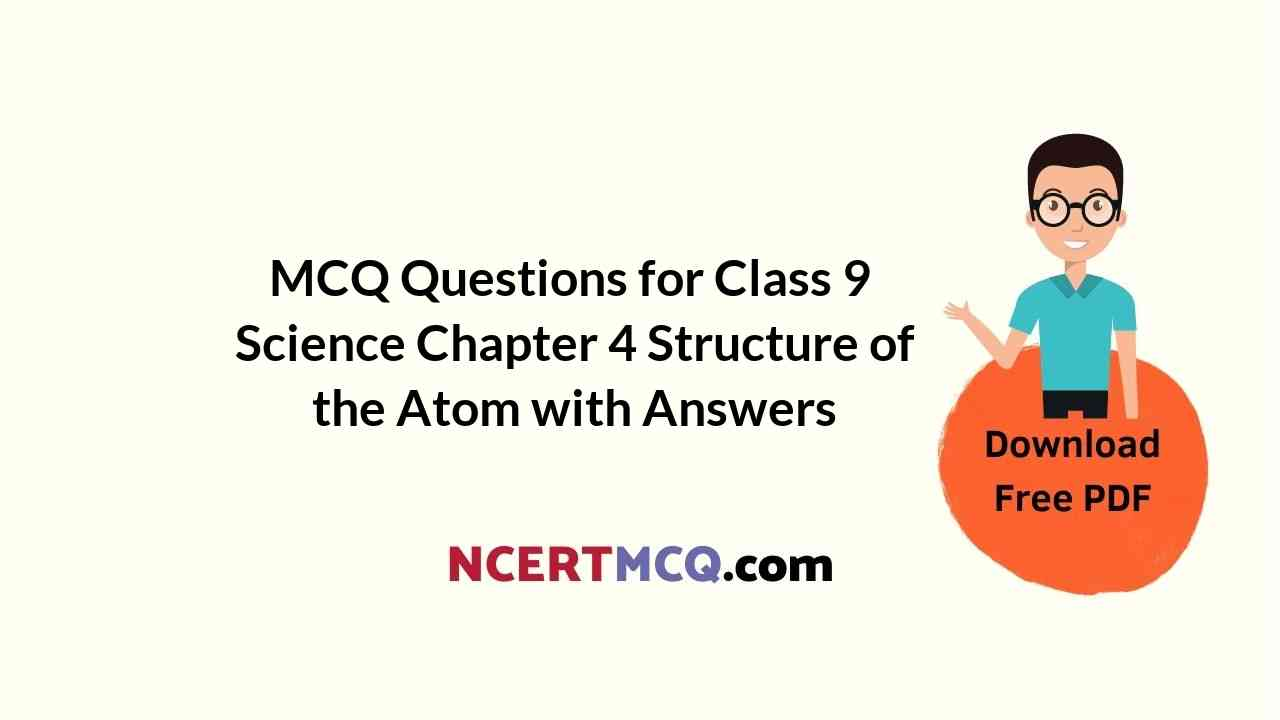Check the below Online Education NCERT MCQ Questions for Class 9 Science Chapter 4 Structure of the Atom with Answers Pdf free download. MCQ Questions for Class 9 Science with Answers were prepared based on the latest exam pattern. We have Provided Structure of the Atom Class 9 Science MCQs Questions with Answers to help students understand the concept very well. https://ncertmcq.com/mcq-questions-for-class-9-science-with-answers/

You can refer to NCERT Solutions for Class 9 Science Chapter 4 Structure of the Atom to revise the concepts in the syllabus effectively and improve your chances of securing high marks in your board exams.

## Online Education for Structure of the Atom Class 9 MCQs Questions with Answers

Structure Of Atom Class 9 MCQ Question 1.
Which of the following correctly represents the electronic distribution in the Mg atom?
(a) 3, 8, 1
(b) 2, 8, 2
(c) 1, 8, 3
(d) 8, 2, 2

Structure Of Atom Class 9 MCQ With Answers Question 2.
Rutherford’s ‘alpha (α) particles scattering experiment’ resulted in the discovery of
(a) electron
(b) proton
(c) nucleus in the atom
(d) atomic mass

Answer: (c) nucleus in the atom

Class 9 Science Chapter 4 MCQ Question 3.
The number of electrons in an element X is 15 and the number of neutrons is 16. Which of the following is the correct representation of the element?
(a) $$_{15}^{31}$$X
(b) $$_{16}^{31}$$X
(c) $$_{15}^{16}$$X
(d) $$_{16}^{15}$$X

Answer: (a) $$_{15}^{31}$$X

MCQ On Structure Of Atom Class 9 Question 4.
Which of the following are true for an element?
(i) Atomic number = number of protons + number of electrons
(ii) Mass number = number of protons + number of neutrons
(iii) Atomic mass = number of protons = number of neutrons
(iv) Atomic number = number of protons = number of electrons
(a) (i) and (ii)
(b) (i) and (iii)
(c) (ii) and (iii)
(d) (ii) and (iv)

Structure Of Atom MCQ Class 9 Question 5.
Atomic models have been improved over the years. Arrange the following atomic models in the order of their chronological order
(i) Rutherford’s atomic model
(ii) Thomson’s atomic model
(ii) Bohr’s atomic model
(a) (i), (ii) and (iii)
(b) (ii), (iii) and (i)
(c) (ii), (i) and (iii)
(d) (iii), (ii) and (i)

Answer: (c) (ii), (i) and (iii)

Class 9 Science Chapter 4 MCQ With Answers Question 6.
The ion of an element has 3 positive charges. Mass number of the atom is 27 and the number of neutrons is 14. What is the number of electrons in the ion?
(a) 13
(b) 10
(c) 14
(d) 16

Class 9 Science Ch 4 MCQ Question 7.
Identify the Mg2+ ion from the figure where, n and p represent the number of neutrons and protons respectively.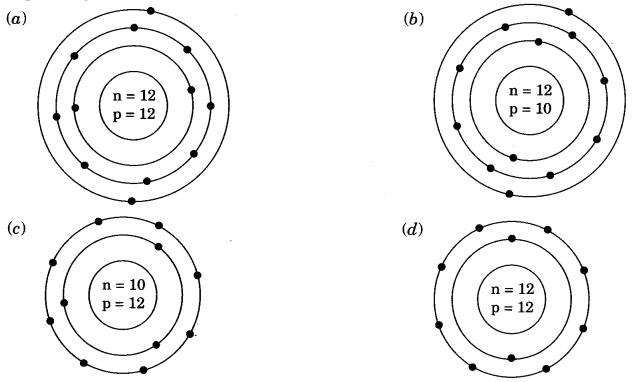MCQ Structure Of Atom Class 9 Question 8.
The first model of an atom was given by
(a) N. Bohr
(b) E. Goldstein
(c) Rutherford
(d) J.J. Thomson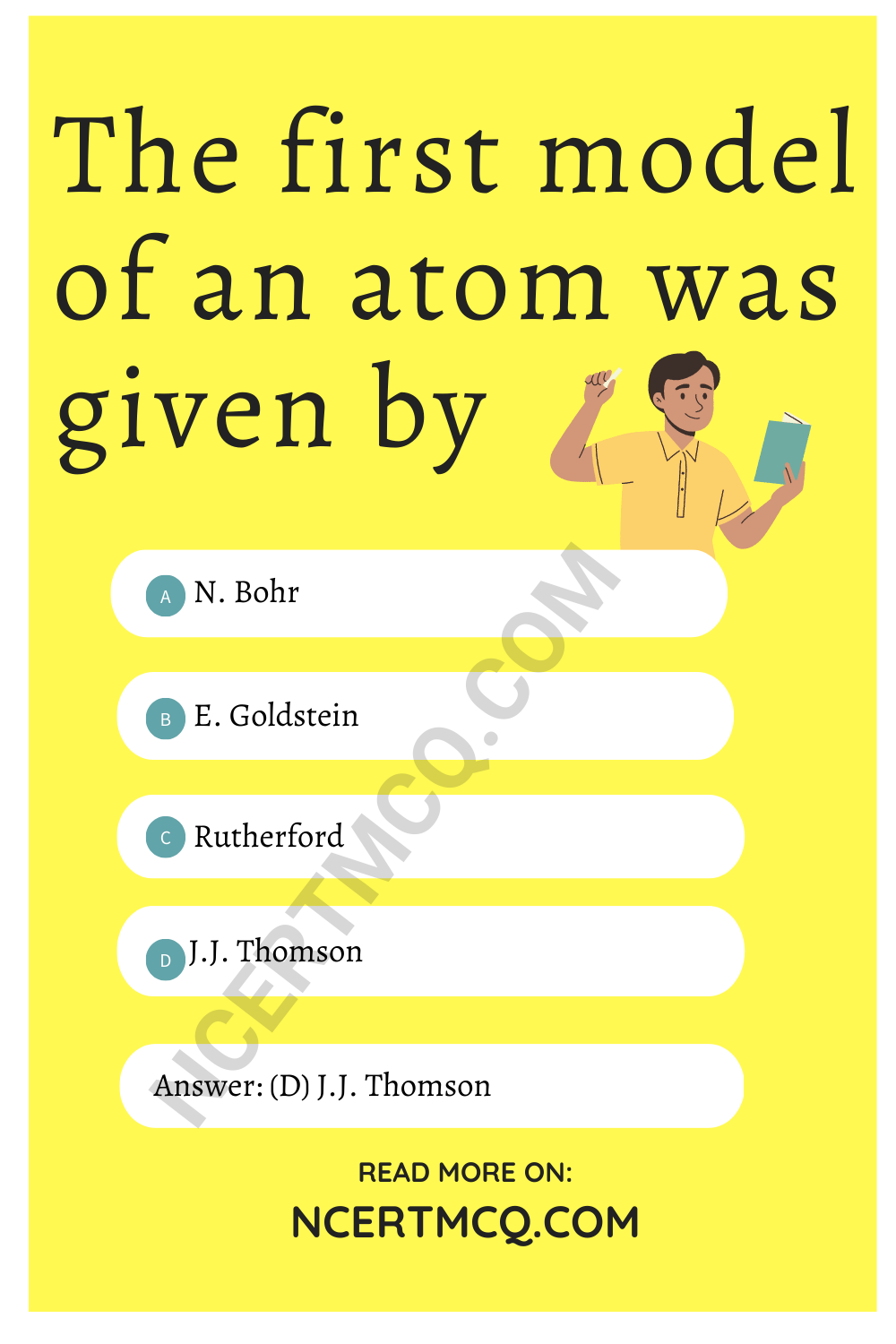Structure Of The Atom Class 9 MCQ Question 9.
An atom with 3 protons and 4 neutrons will have a valency of
(a) 3
(b) 7
(c) 1
(d) 4

Class 9 Structure Of Atom MCQ Question 10.
Which of the following statement is always correct?
(a) An atom has equal number of electrons and protons.
(b) An atom has equal number of electrons and neutrons.
(c) An atom has equal number of protons and neutrons.
(d) An atom has equal number of electrons, protons and neutrons.

Answer: (a) An atom has equal number of electrons and protons.

MCQ On Structure Of Atom Class 9 Question 11.
Which of the following statements about Rutherford’s model of atom are correct?
(i) Considered the nucleus as positively charged.
(ii) Established that the a-particles are four times as heavy as a hydrogen atom.
(iii) Can be compared to solar system.
(iv) Was in agreement with Thomson’s model.
(a) (i) and (iii)
(b) (ii) and (iii)
(c) (i) and (iv)
(d) only (i)

MCQ Questions For Class 9 Science Chapter 4 Question 12.
Hydrogen exists in three isotopic forms, $$_{1}^{1}$$H, $$_{1}^{2}$$H, $$_{1}^{3}$$H known as protium, deuterium and tritium. Why are all the isotopes neutral in nature?
(a) Since neutrons are neutral in nature hence isotopes are electrically neutral.
(b) All the isotopes have one electron and one proton, hence they are neutral.
(c) All the isotopes have one proton and one neutron, hence they are neutral.
(d) Increasing number of protons in the isotopes make them neutral.

Answer: (b) All the isotopes have one electron and one proton, hence they are neutral.

Ch 4 Science Class 9 MCQ Question 13.
How many electrons are present in M-shell of an element with atomic number 20?
(a) 5
(b) 8
(c) 12
(d) 18

Chapter 4 Science Class 9 MCQ Question 14.
There are two atomic species X and Y, such thatWhich of the following statements is true about X and Y?
(a) X and Y are isobars.
(b) X and Y have different chemical properties.
(c) X and Y have different physical properties.
(d) All of these.

Answer: (c) X and Y have different physical properties.

MCQ Of Structure Of Atom Class 9 Question 15.
The ion of an element has 3 positive charges. Mass number of the atom is 27 and the number of neutrons is 14. What is the number of electrons in the ion?
(a) 13
(b) 10
(c) 14
(d) 16

Question 16.
An alpha particle is also known as:
(a) subatomic particle
(b) an unionised helium atom
(c) a neutral particle
(d) a doubly-charged helium ion

Answer: (d) a doubly-charged helium ion

Question 17.
There are three isotopes of carbon which are named as C-12, C-13 and C-14 out of which C-12 is the most abundant isotope. In the given structures of 3 isotopes, what will be the composition of the nucleus?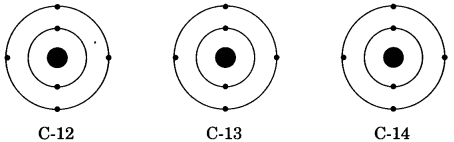(a) C-12 : 6p + 6n, C-13 : 7p + 6n, C-14 : 8p + 6n
(b) C-12 : 6p + 6n, C-13 : 6p + 7n, C-14 : 6p + 8n
(c) C-12 : 6p + 6n, C-13 : 5p + 8n, C-14 : 7p + 7n
(d) C-12 : 6p + 6n, C-13 : 12p + 1n, C-14 : 5p + 9n

Answer: (b) C-12 : 6p + 6n, C-13 : 6p + 7n, C-14 : 6p + 8n

Question 18.
The charge on an electron is equal to:
(a) 1.6 × 10-19 C of -ve charge
(b) 2.6 × 10-19 C of -ve charge
(c) 1.6 × 10-22 C of -ve charge
(d) 1.6 × 10-23 C of -ve charge

Answer: (a) 1.6 × 10-19 C of -ve charge

Question 19.
Which of the following isotope is used in the treatment of blood cancer?
(a) P-32
(b) I-131
(c) Co-60
(d) any of these

Question 20.
Isobars do not differ in the number of
(a) protons
(b) electrons
(c) neutrons
(d) nucleonsFill in the blanks

1. Rutherford’s a-particle scattering experiment led to the discovery of the ……………….

2. Isotopes have same ……………… but different ……………..

3. Neon and chlorine have atomic numbers 10 and 17 respectively. Their valencies will be ………….. and ……………. respectively.

4. The electronic configuration of silicon is …………… and that of sulphur is ……………..

Answer: 2, 8, 4, and 2, 8, 6

5. Cathode rays consist of negatively charged particles known as ………………

6. ……………. are the atoms containing same number of neutrons.

7. The outermost shell of an atom is known as its ……………..

8. Alpha particles are helium nuclei (He2+) and have …………. mass and ……………. units charge.

9. The maximum number of electrons in a shell is given by ……………..

10. Neutrons were discovered by …………….

Match the names of the scientists given the columns A with their contributions towards the understanding of the atomic structure as given in column B.

1.

 Column A Column B (a) Ernest Rutherford (i) Indivisibility of atoms (b) J. J. Thomson (ii) Stationary orbits (c) Dalton (iii) Concept of nucleus (d) Neils Bohr (iv) Discovery of electrons (e) James Chadwick (v) Atomic number (f) E. Goldstein (vi) Neutron (g) Mosley (vii) Canal rays

 Column A Column B (a) Ernest Rutherford (iii) Concept of nucleus (b) J. J. Thomson (iv) Discovery of electrons (c) Dalton (i) Indivisibility of atoms (d) Neils Bohr (ii) Stationary orbits (e) James Chadwick (vi) Neutron (f) E. Goldstein (vii) Canal rays (g) Mosley (v) Atomic number

2.

 Column A Column B (a) Ions (i) Same mass number, different atomic numbers. (b) Isobars (ii) Same number of neutrons, different mass numbers. (c) Isotopes (iii) Formed by loss or gain of electron (d) Isotones (iv) Combining capacity of an atom. (e) Valency (v) Same atomic numbers, different mass numbers.

 Column A Column B (a) Ions (iii) Formed by loss or gain of electron (b) Isobars (i) Same mass number, different atomic numbers. (c) Isotopes (v) Same atomic numbers, different mass numbers. (d) Isotones (ii) Same number of neutrons, different mass numbers. (e) Valency (iv) Combining capacity of an atom.

3.

 Column A Column B Chlorine atom 2, 8 Sodium atom 2, 8, 7 Magnesium ion 2, 8, 2 Magnesium atom 2, 8, 1

 Column A Column B Chlorine atom 2, 8 Sodium atom 2, 8, 7 Magnesium ion 2, 8, 2 Magnesium atom 2, 8, 1

Answer the questions in one word:

1. Which symbols are used to represent different Bohr’s orbits?

2. Which isotope is used in the treatment of cancer?

3. What is the charge of anode rays?

4. There are 14 protons and 13 neutrons in the nucleus of an atom. What is its mass number?

5. Indicate the number of electrons, protons and neutrons in the element $$_{19}^{39}$$K.

Answer: e = 19, p = 19, n = 20

6. The atomic number of cation M2+ is 12. How many electrons are present in it?

7. Which isotope of hydrogen is called protium?

Answer: $$_{1}^{1}$$H

8. Which particles determine the mass of an atom?

Solve the crossword with the help of clues:

Across:
1. Part of atom responsible for mass.
3. Same mass number of species.
5. a-particle scattering experiment.
7. No. of valence electron in sodium.
Down:
2. Discovered the neutron.
4. Latest atomic model given by.
6. Negatively charged subatomic particle.
8. Positively charged substance particle.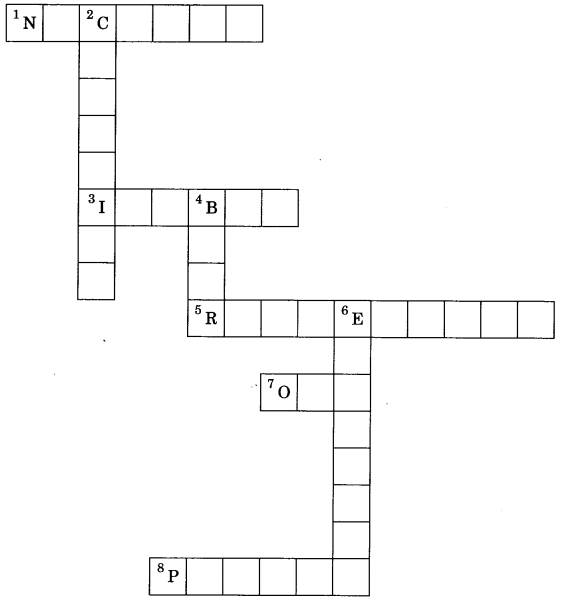Across:
1. Nucleus
3. Isobar
5. Rutherford
7. One

Down: